# RS Aggarwal Solutions for Class 9 Chapter 4: Linear Equations in Two Variables Exercise 4B

## RS Aggarwal Solutions for Class 9 Maths Exercise 4B PDF

RS Aggarwal Solutions for Class 9 Maths can be used as a tool by the students to improve their performance in Mathematics exam. By keeping in mind the difficulties faced by the students while preparing for the exams, our experienced faculties prepare solutions according to their grasping abilities. These solutions are mainly prepared according to present CBSE syllabus with ample number of examples to be practised before starting each exercise. RS Aggarwal Solutions for Class 9 Maths Chapter 4 Linear Equations in Two Variables Exercise 4B are provided here.

## RS Aggarwal Solutions for Class 9 Chapter 4: Linear Equations in Two Variables Exercise 4B Download PDF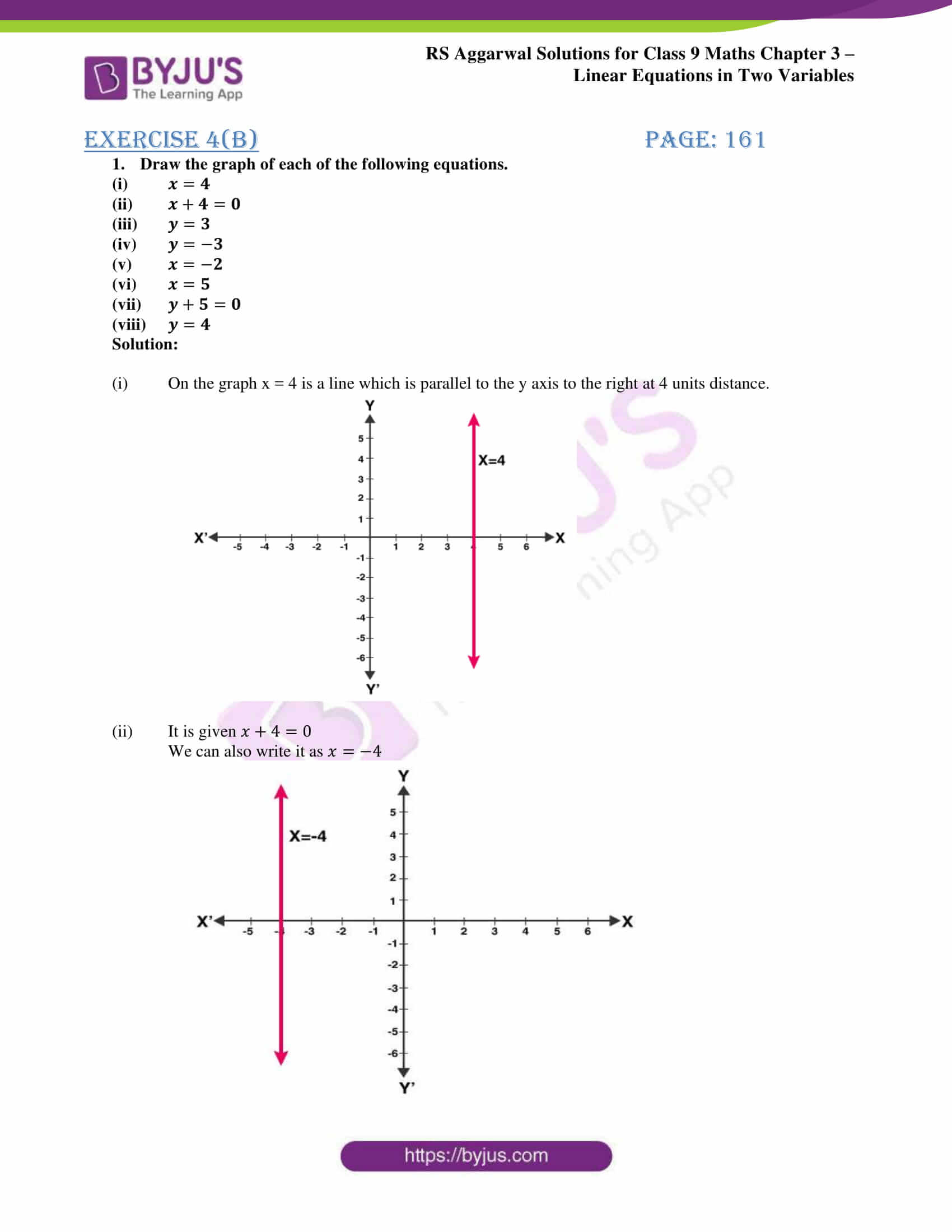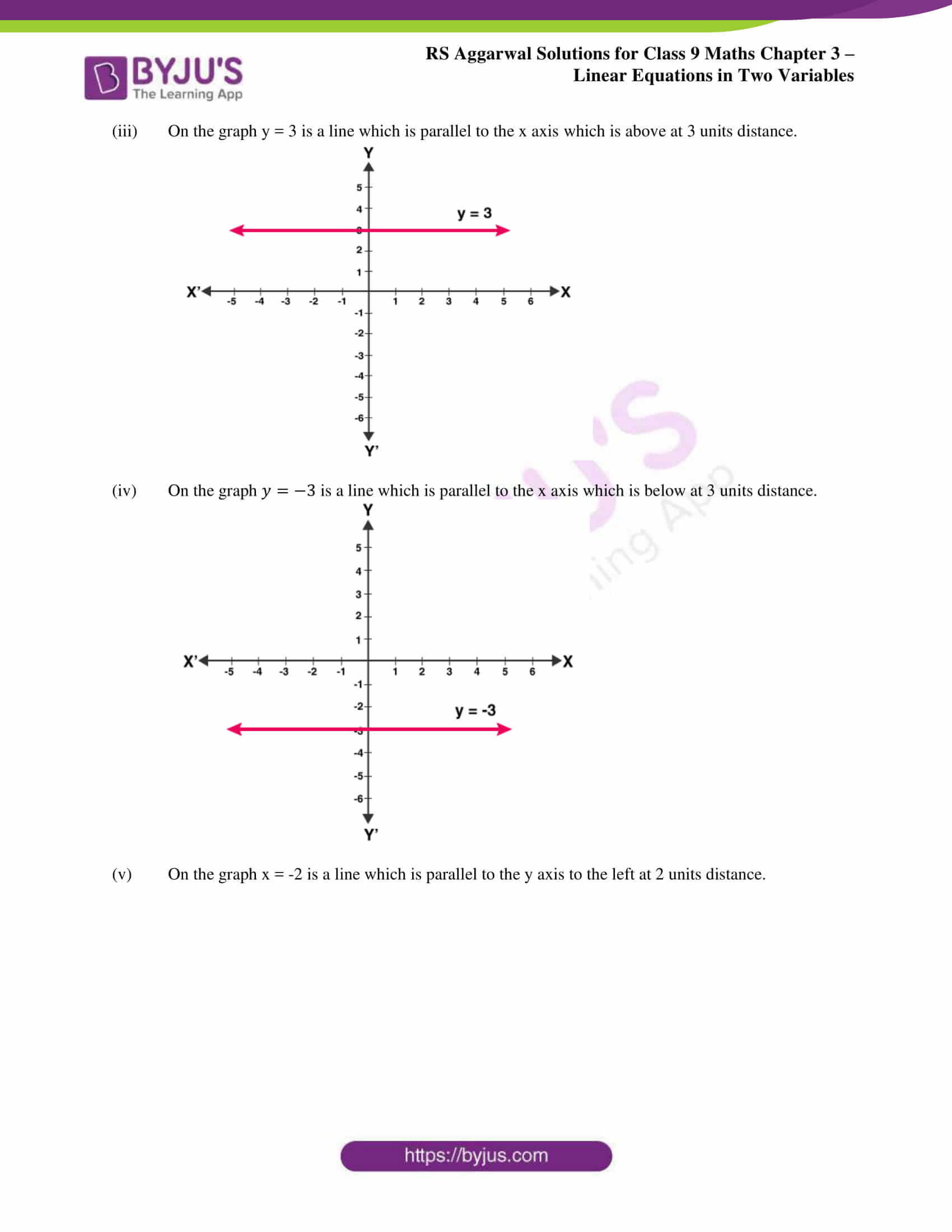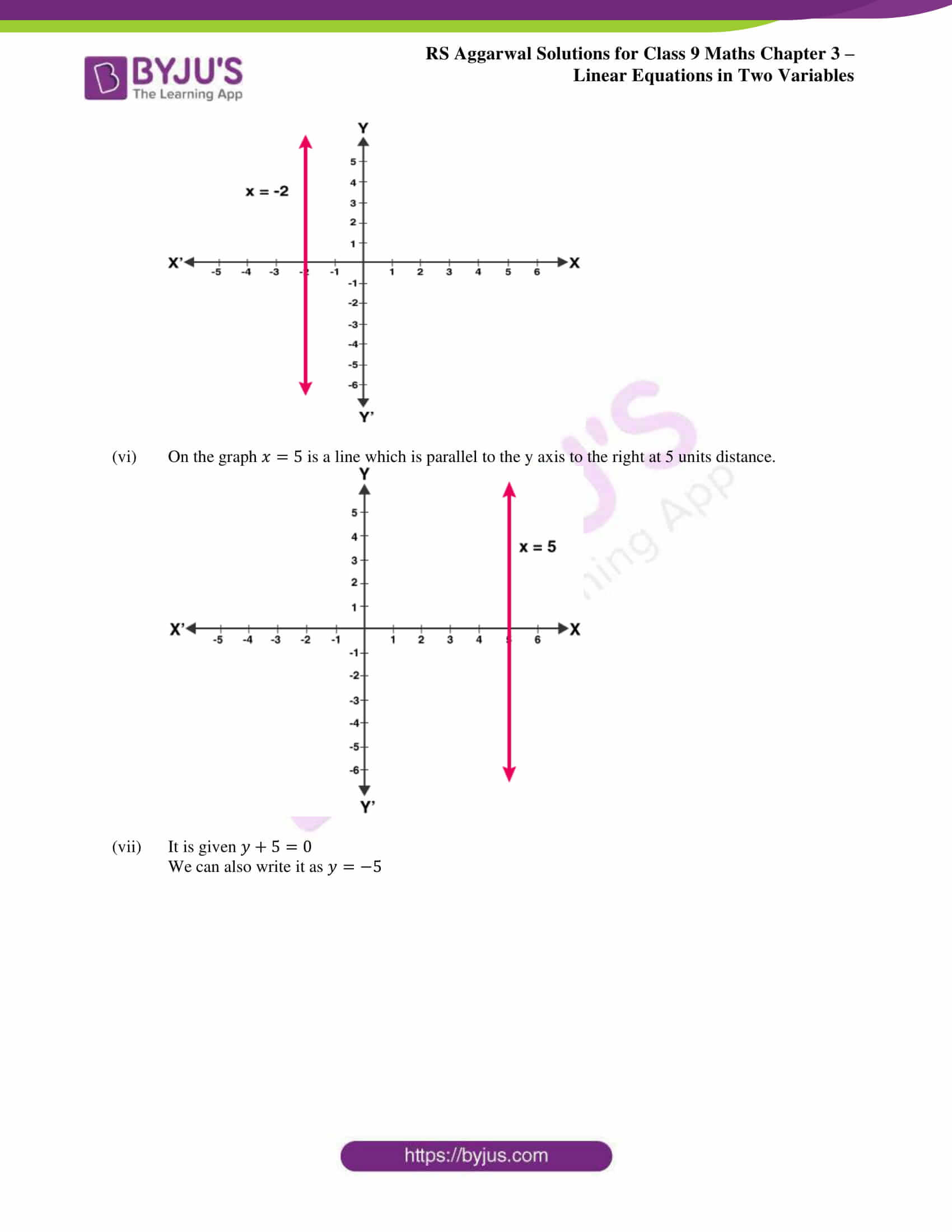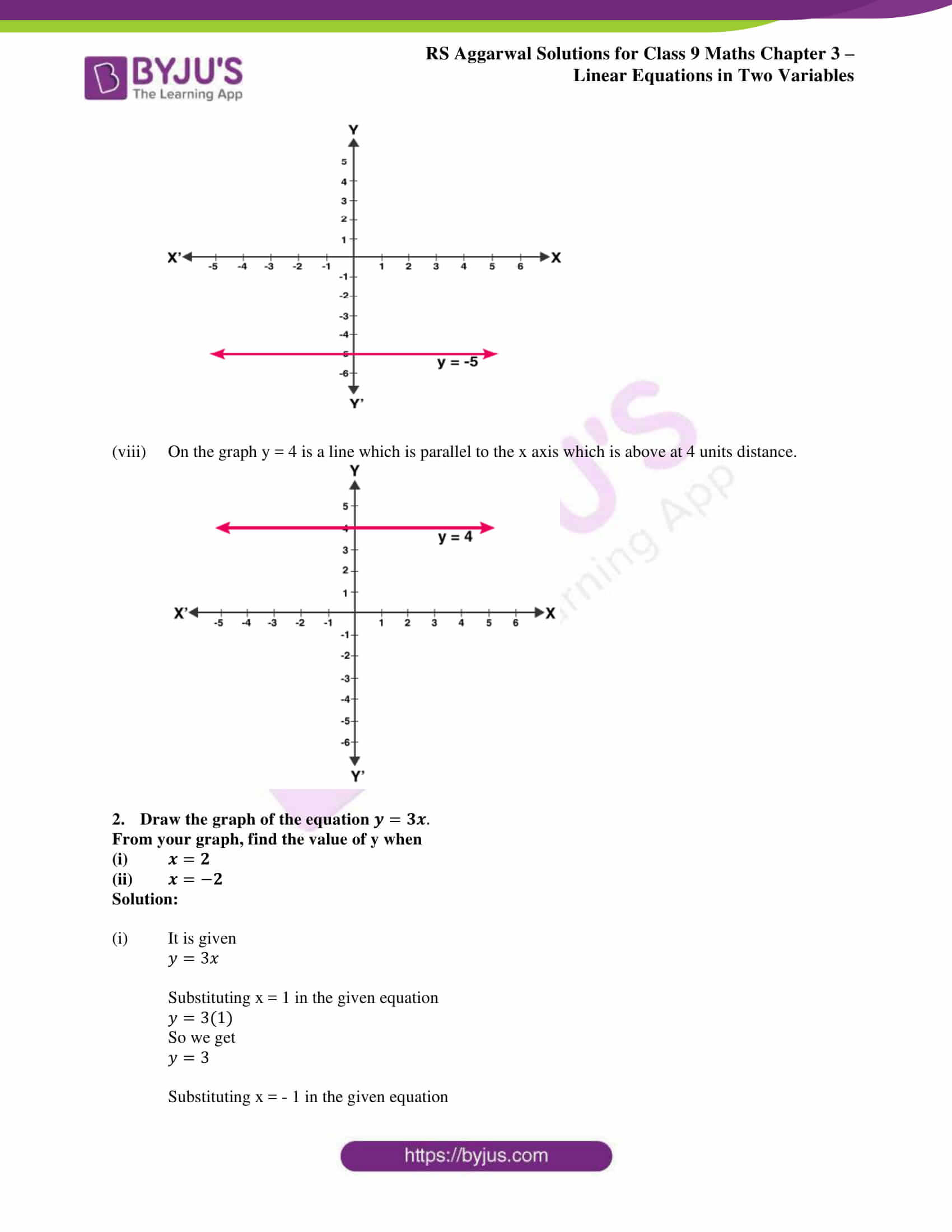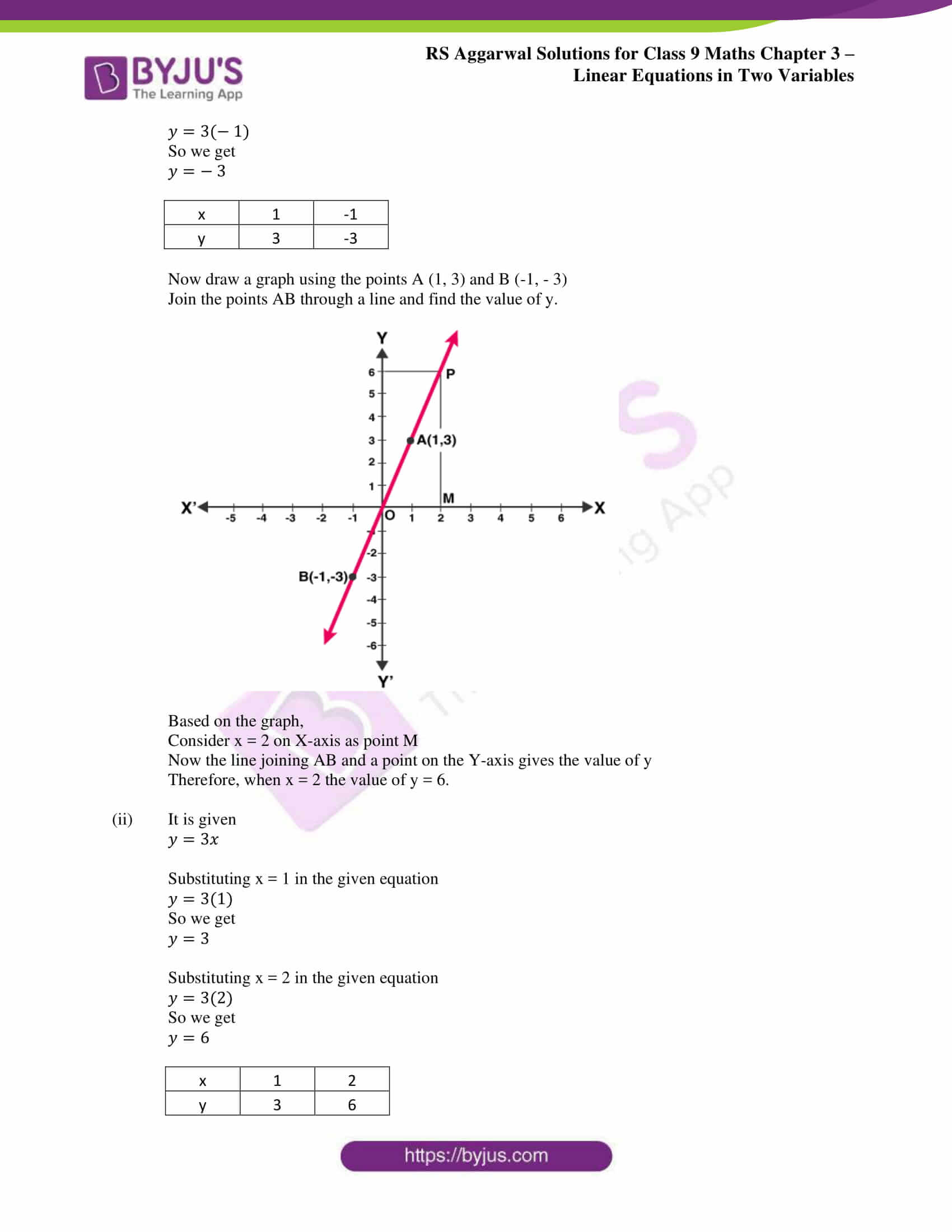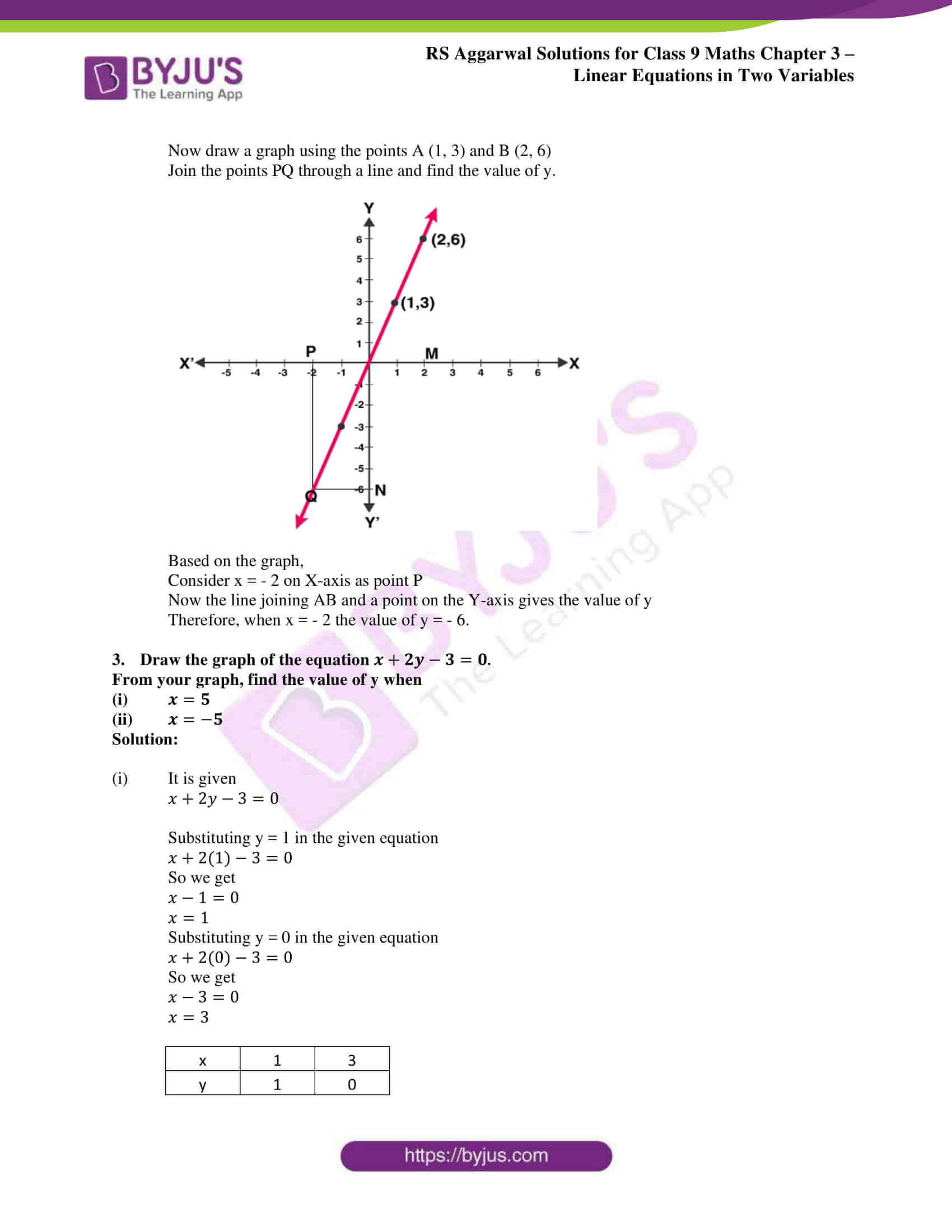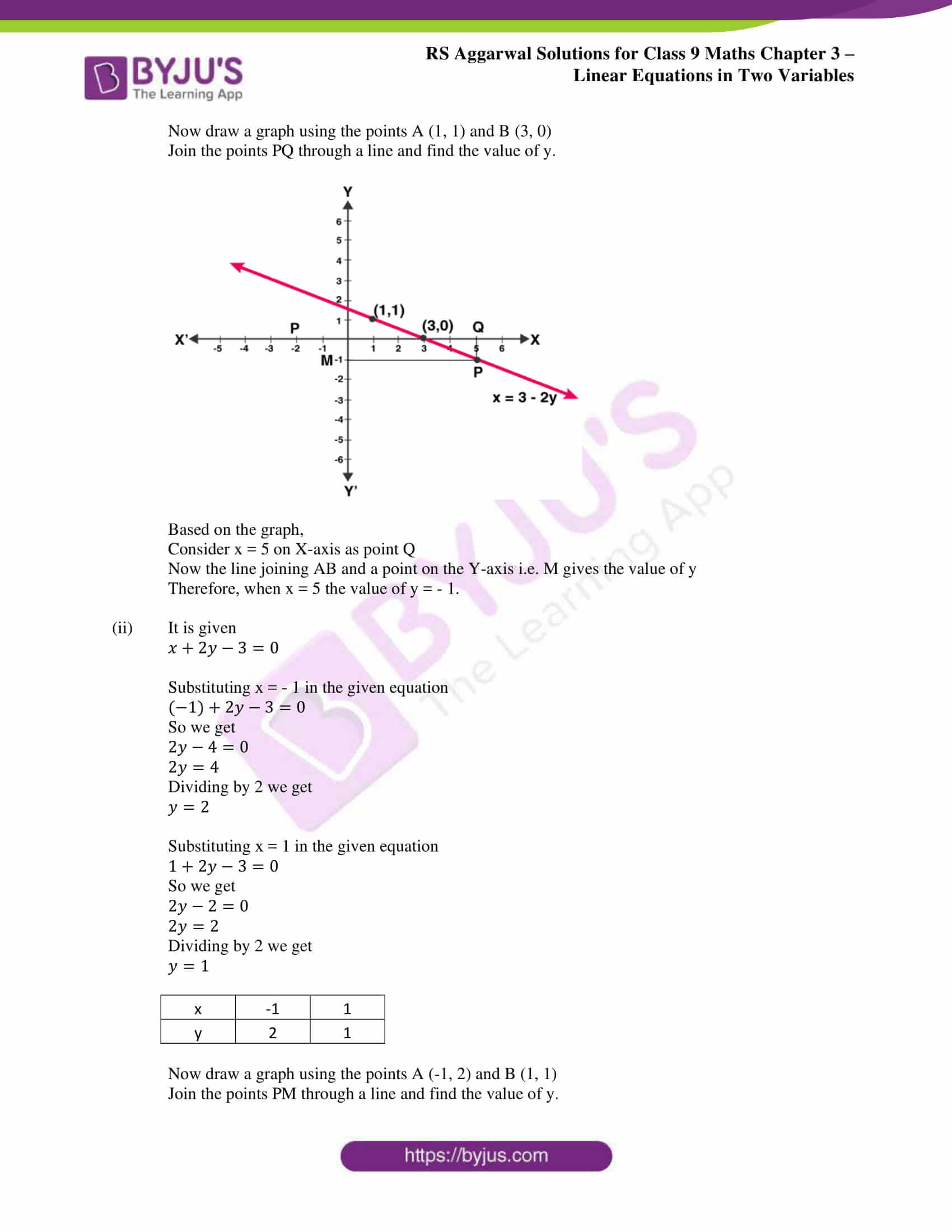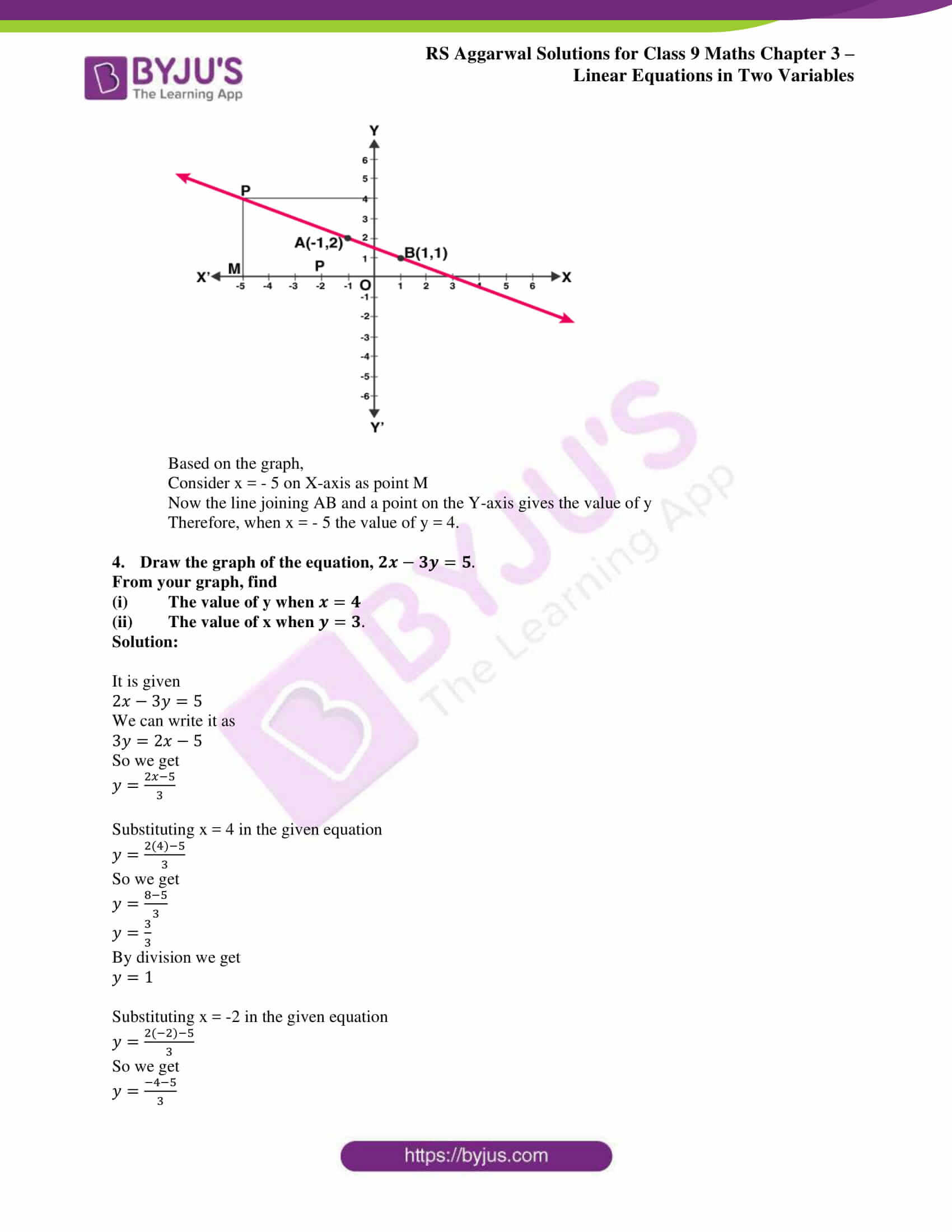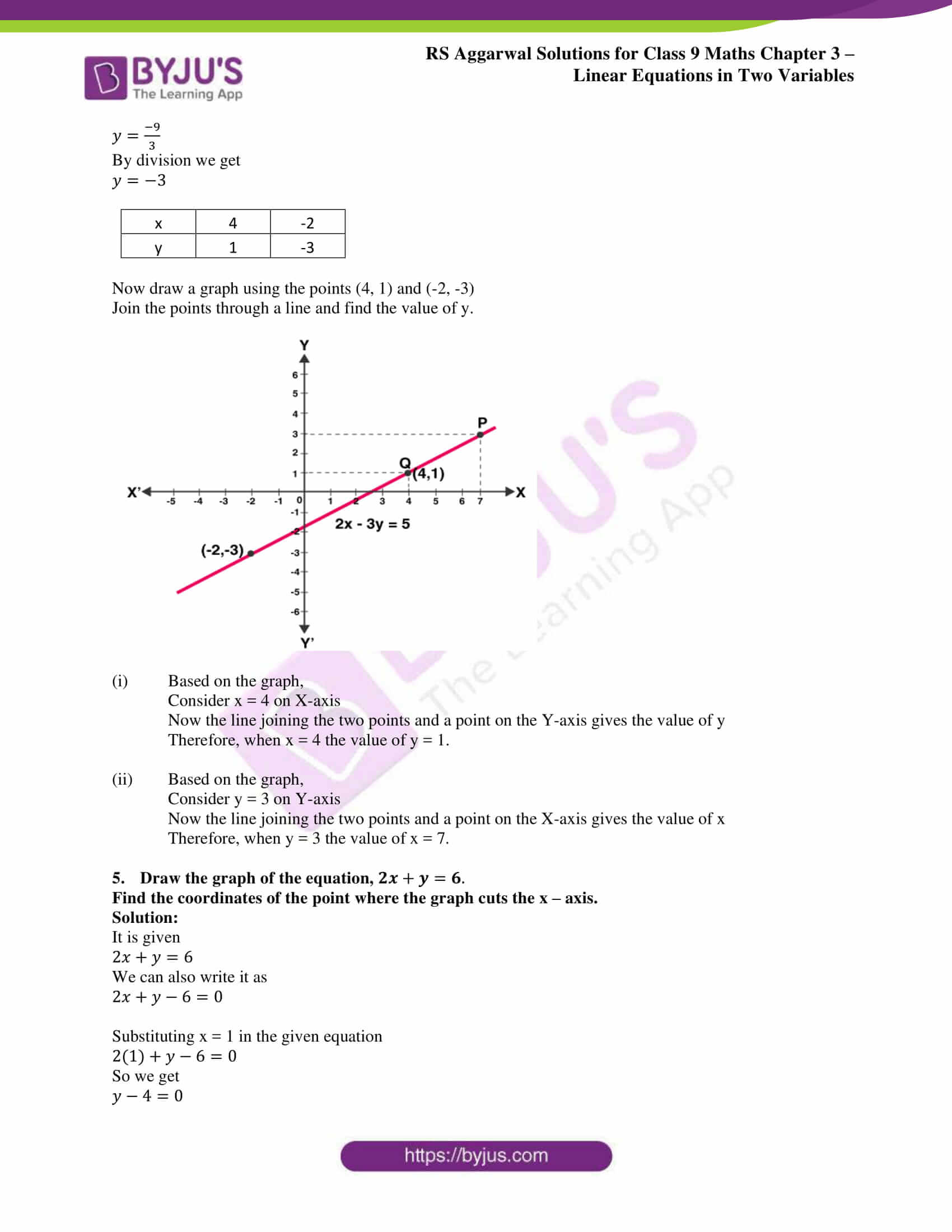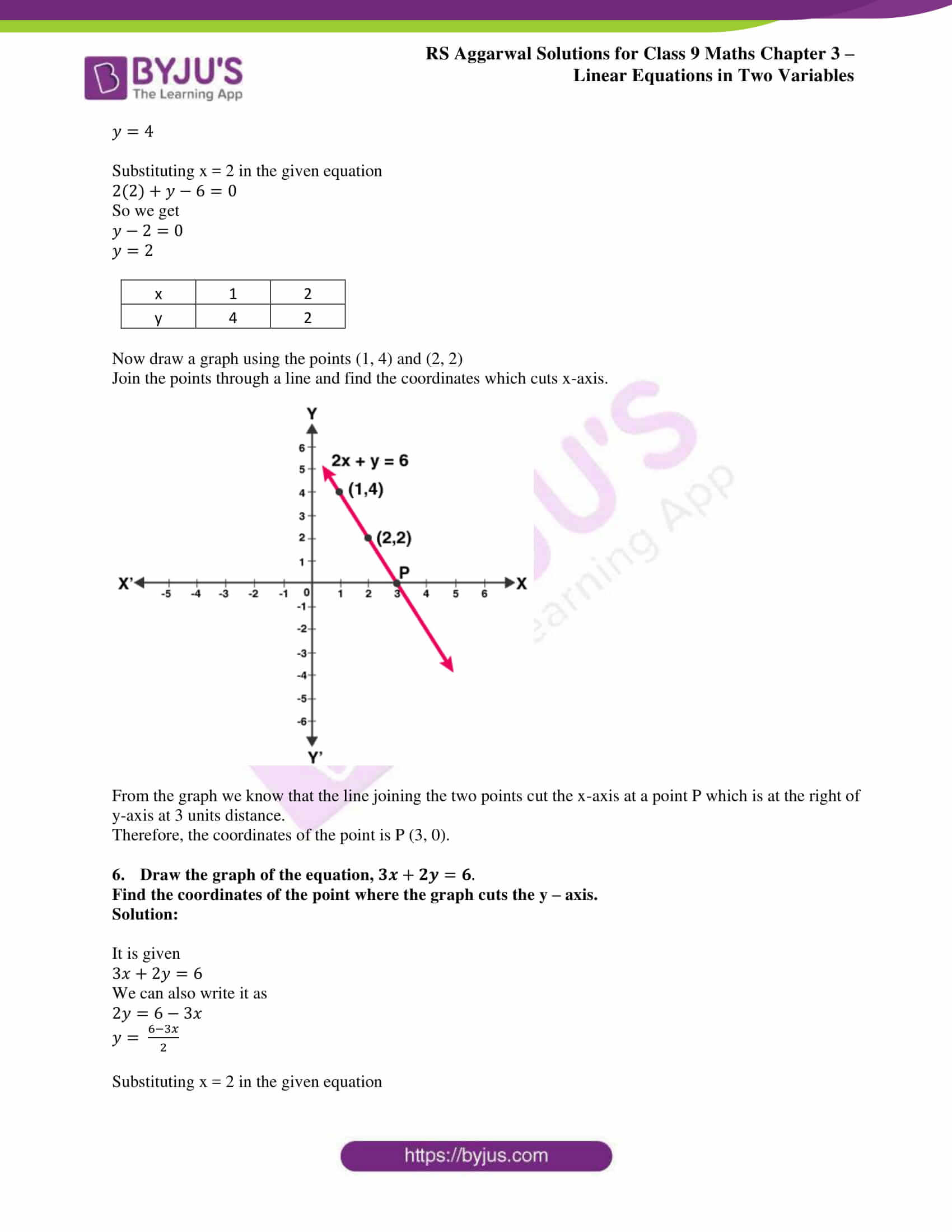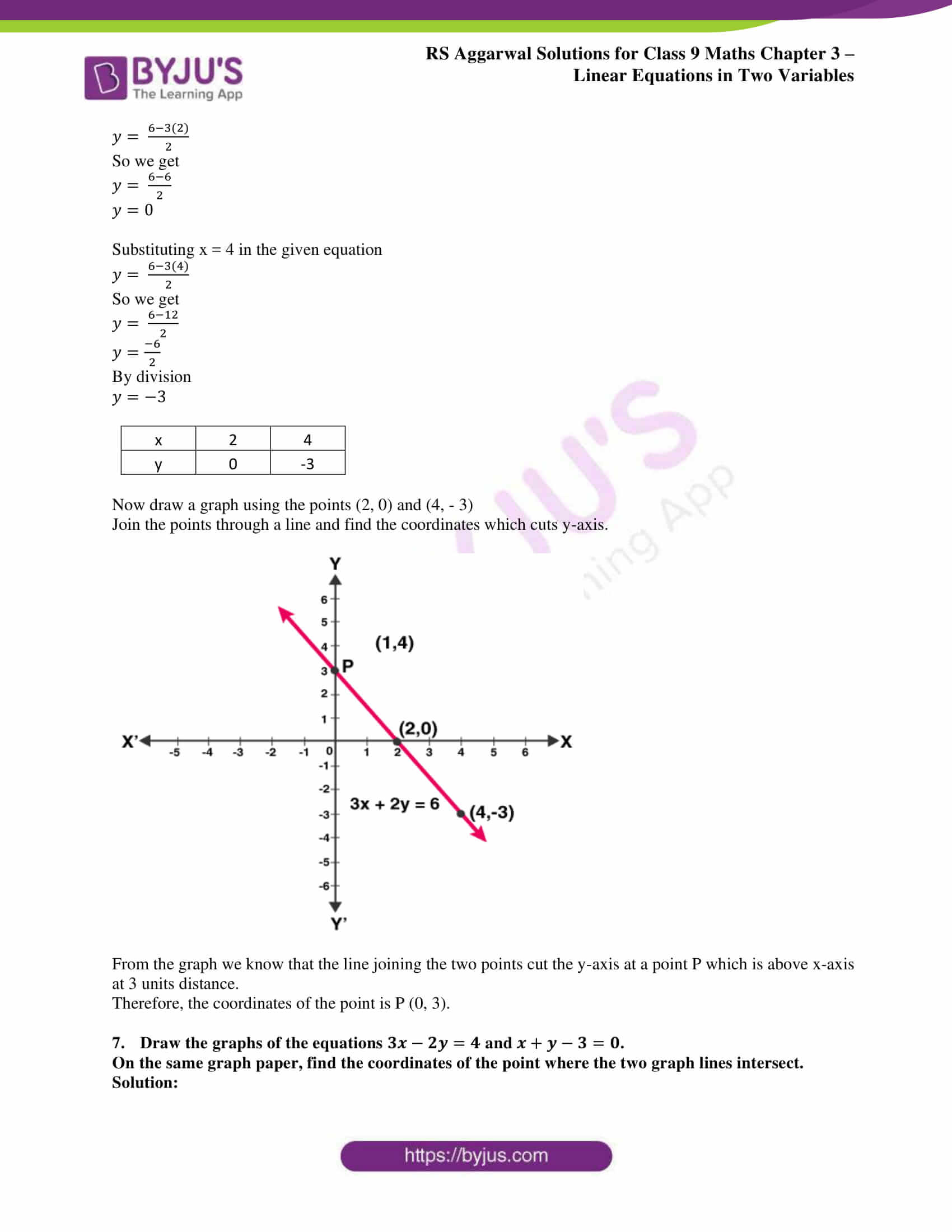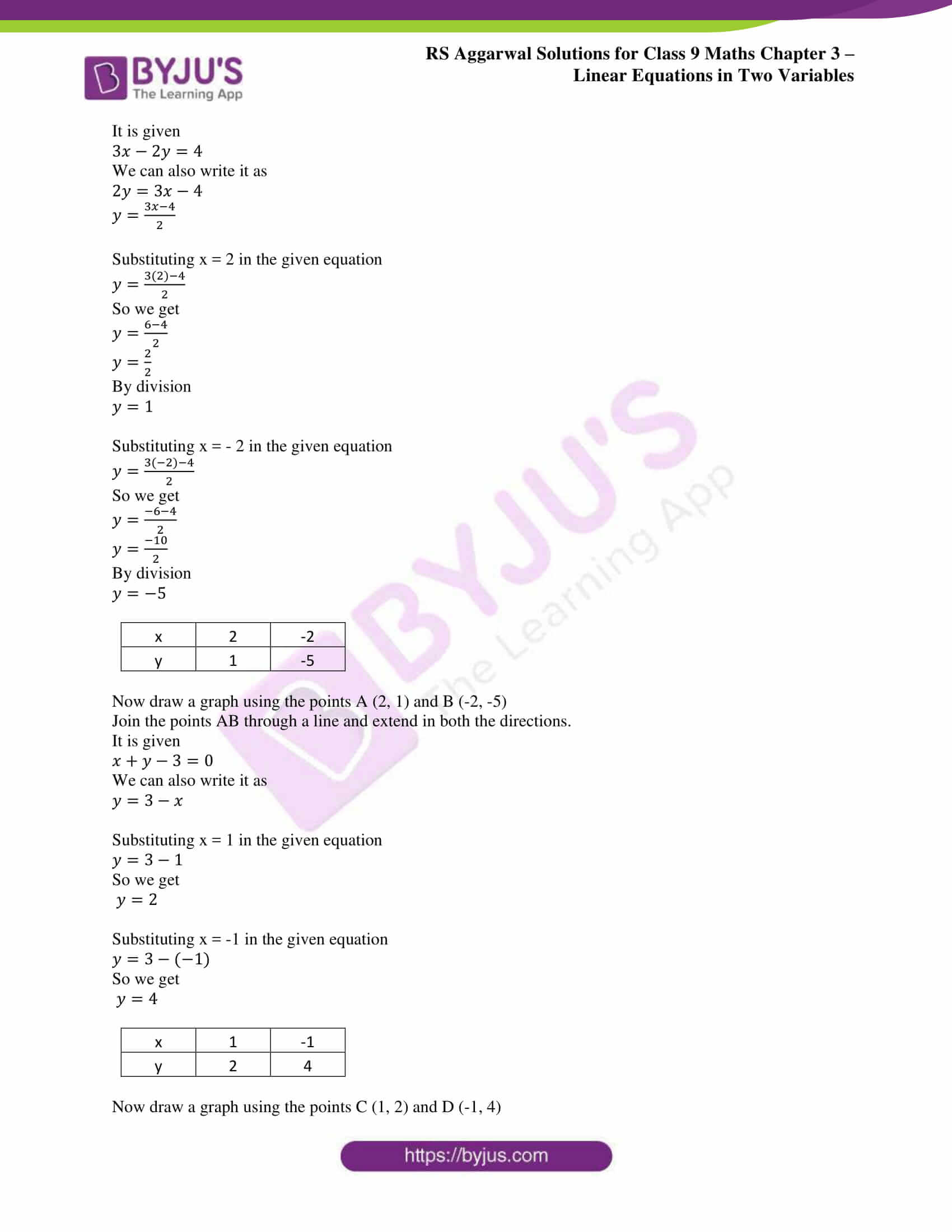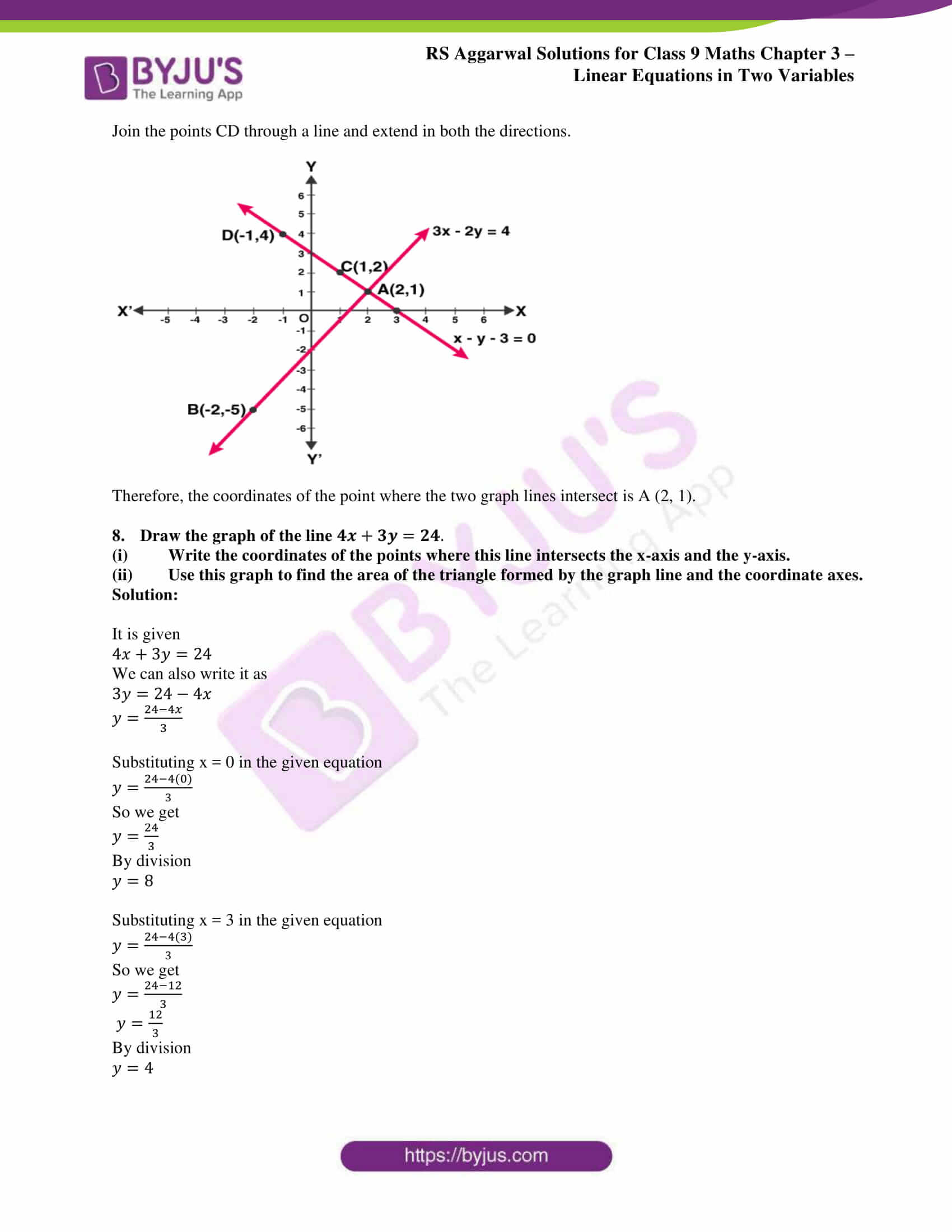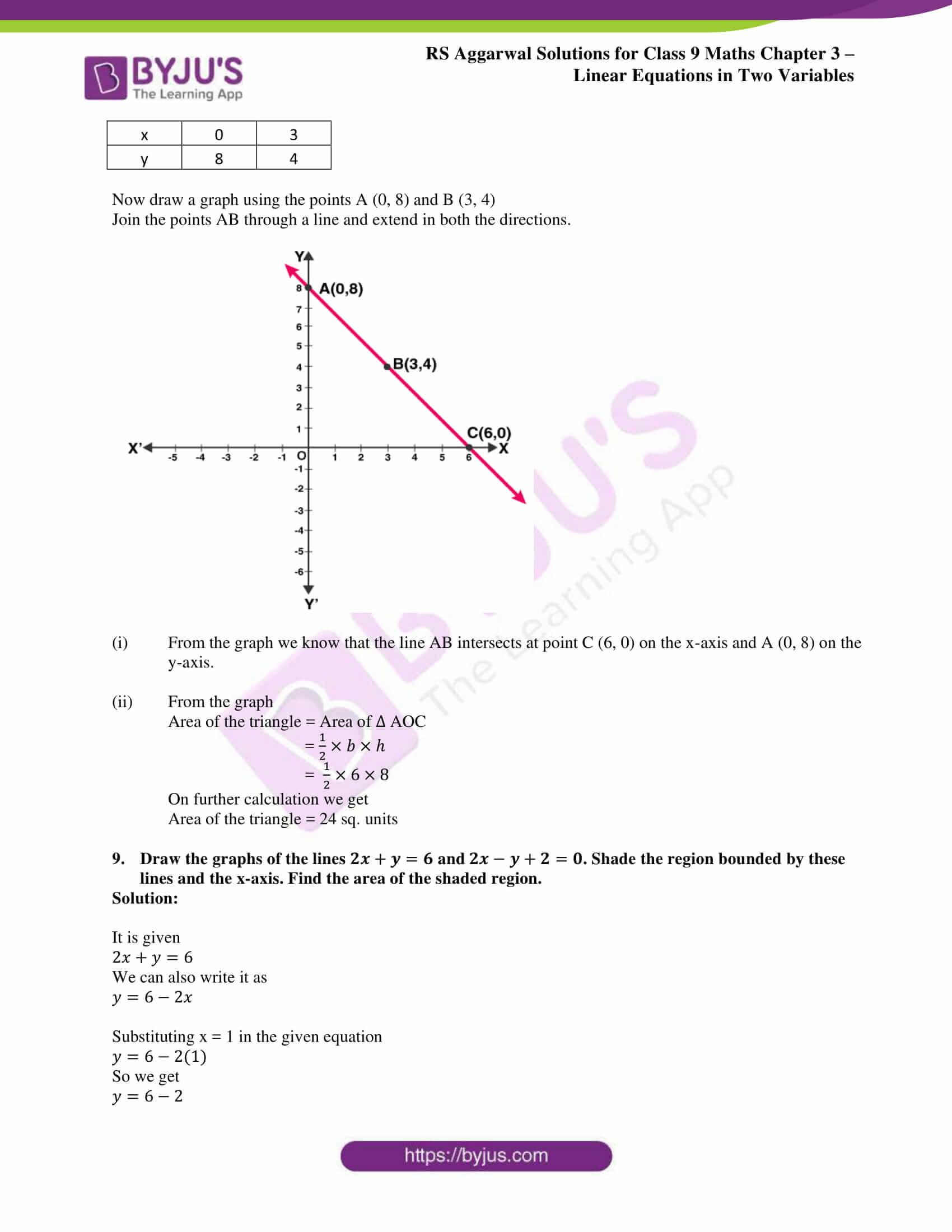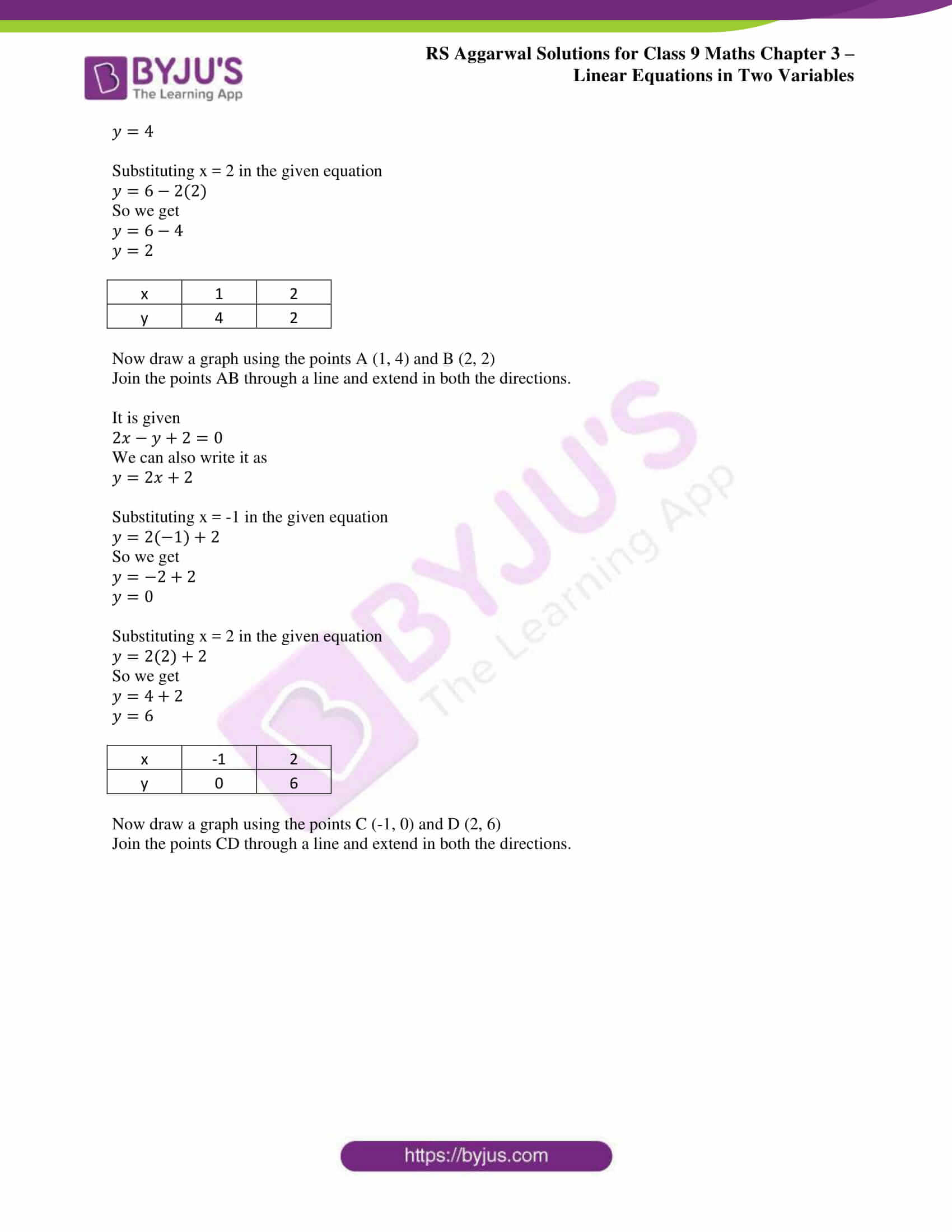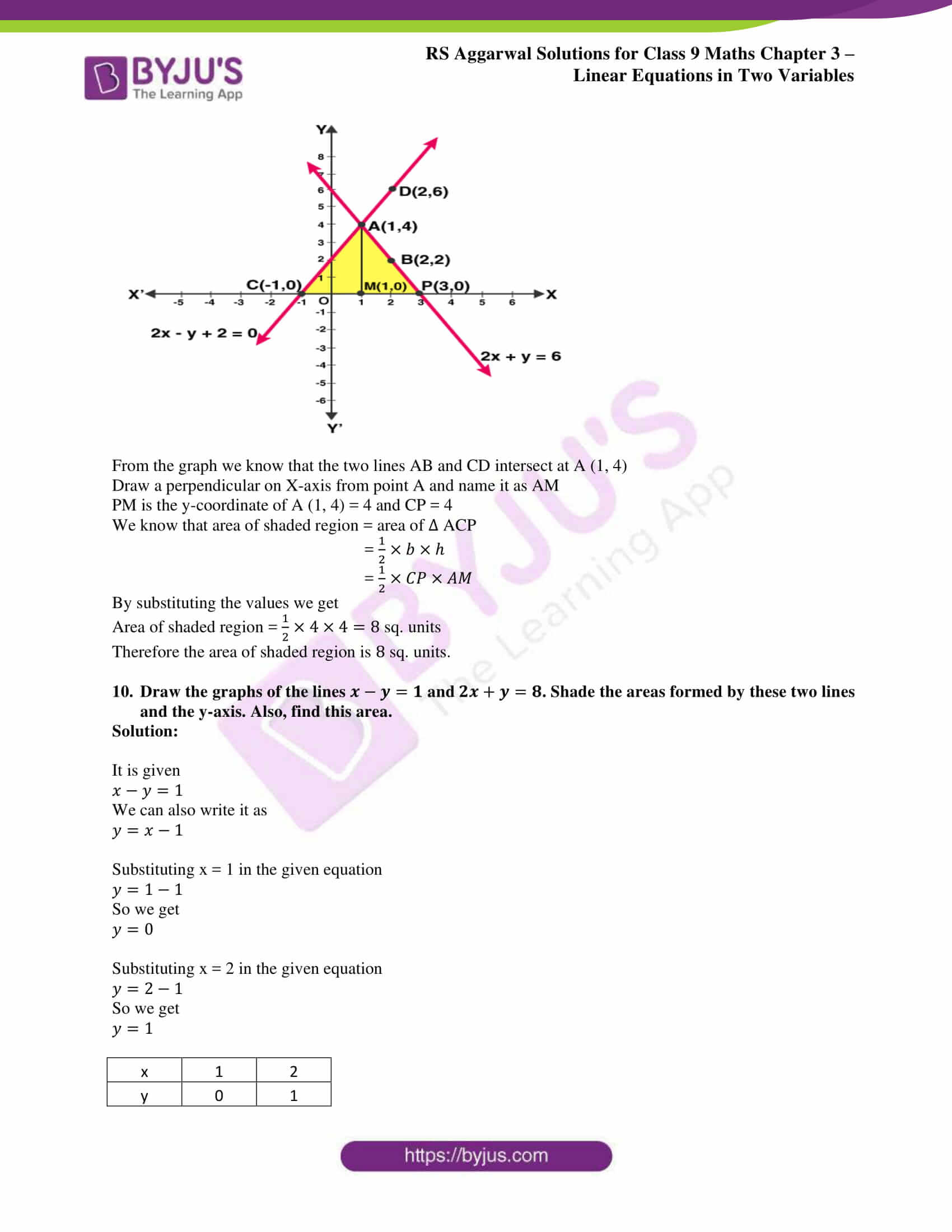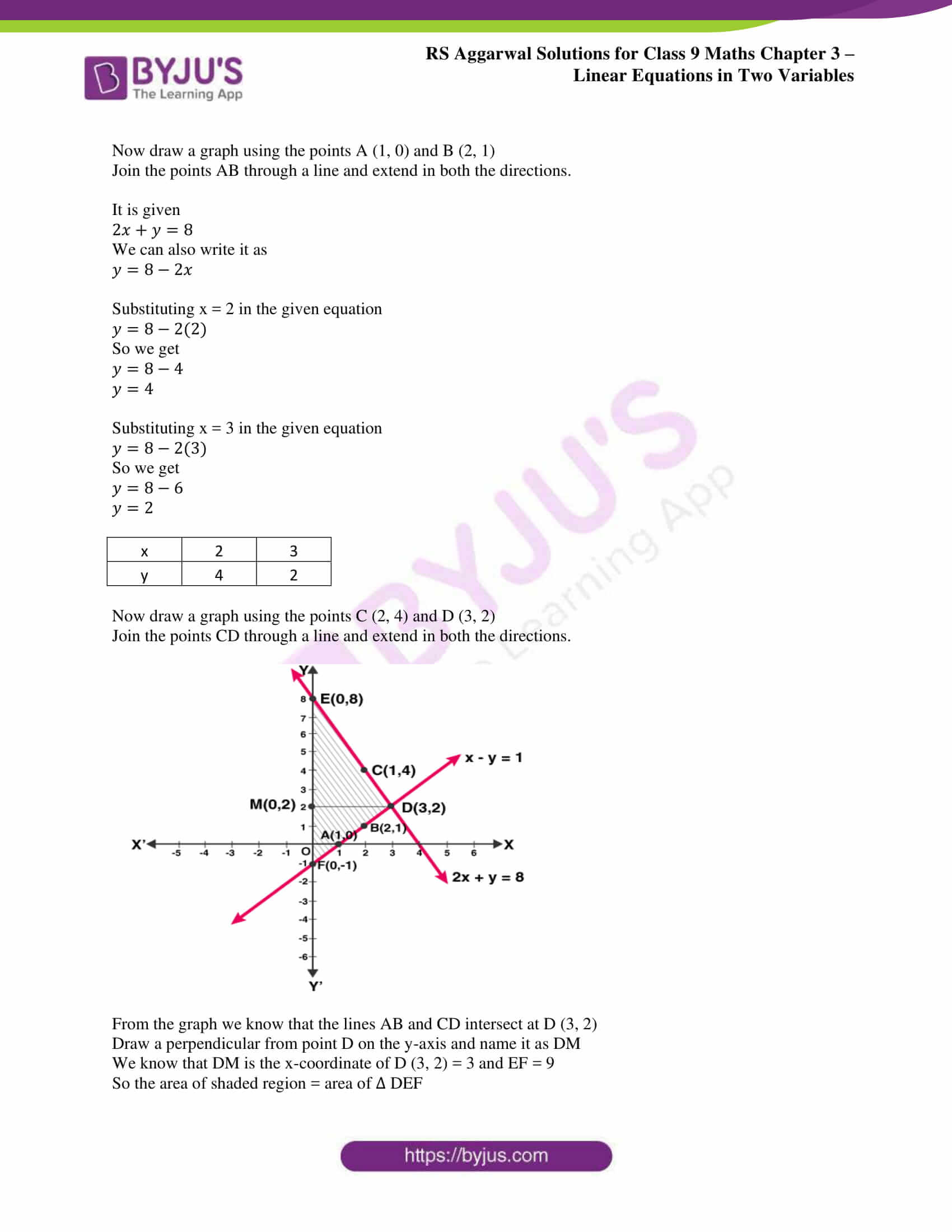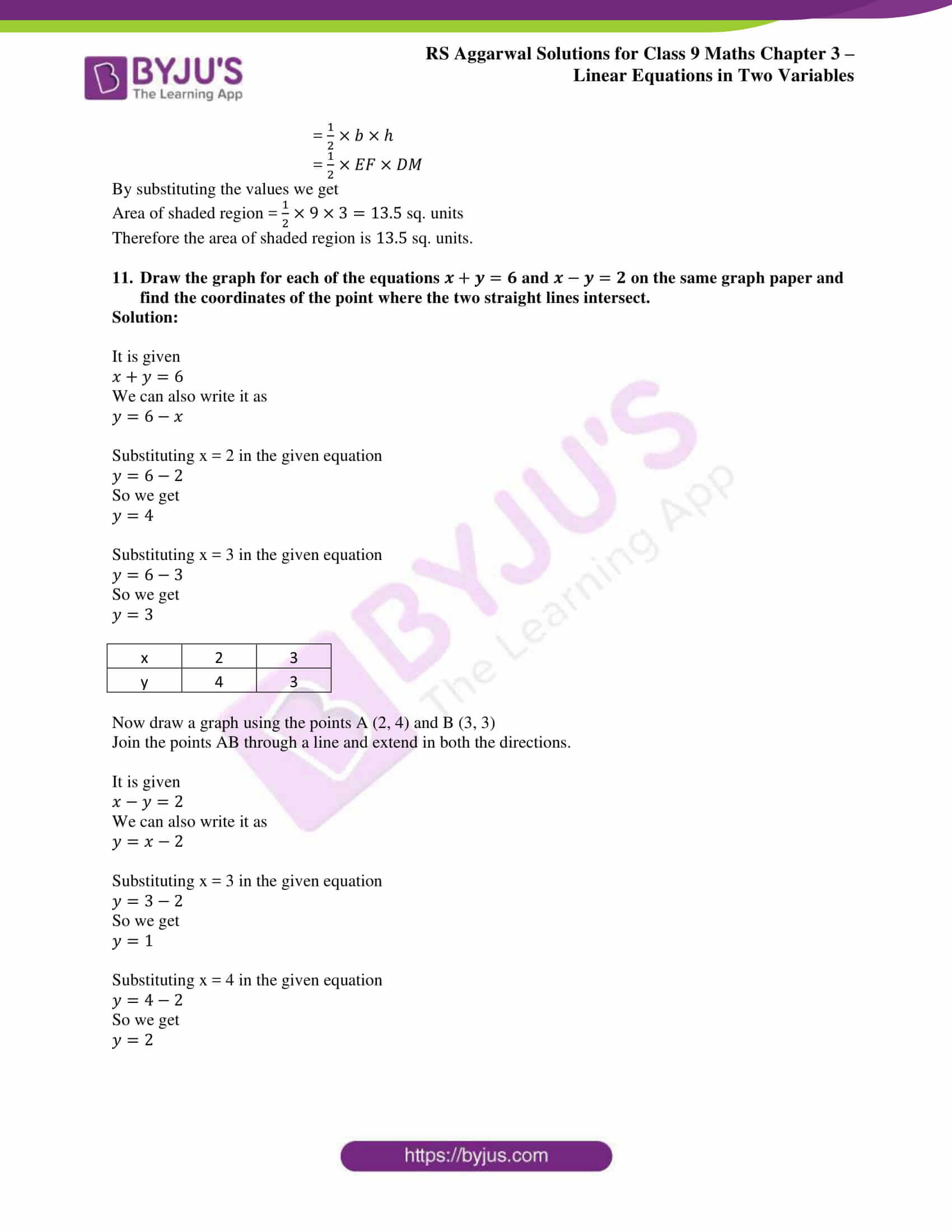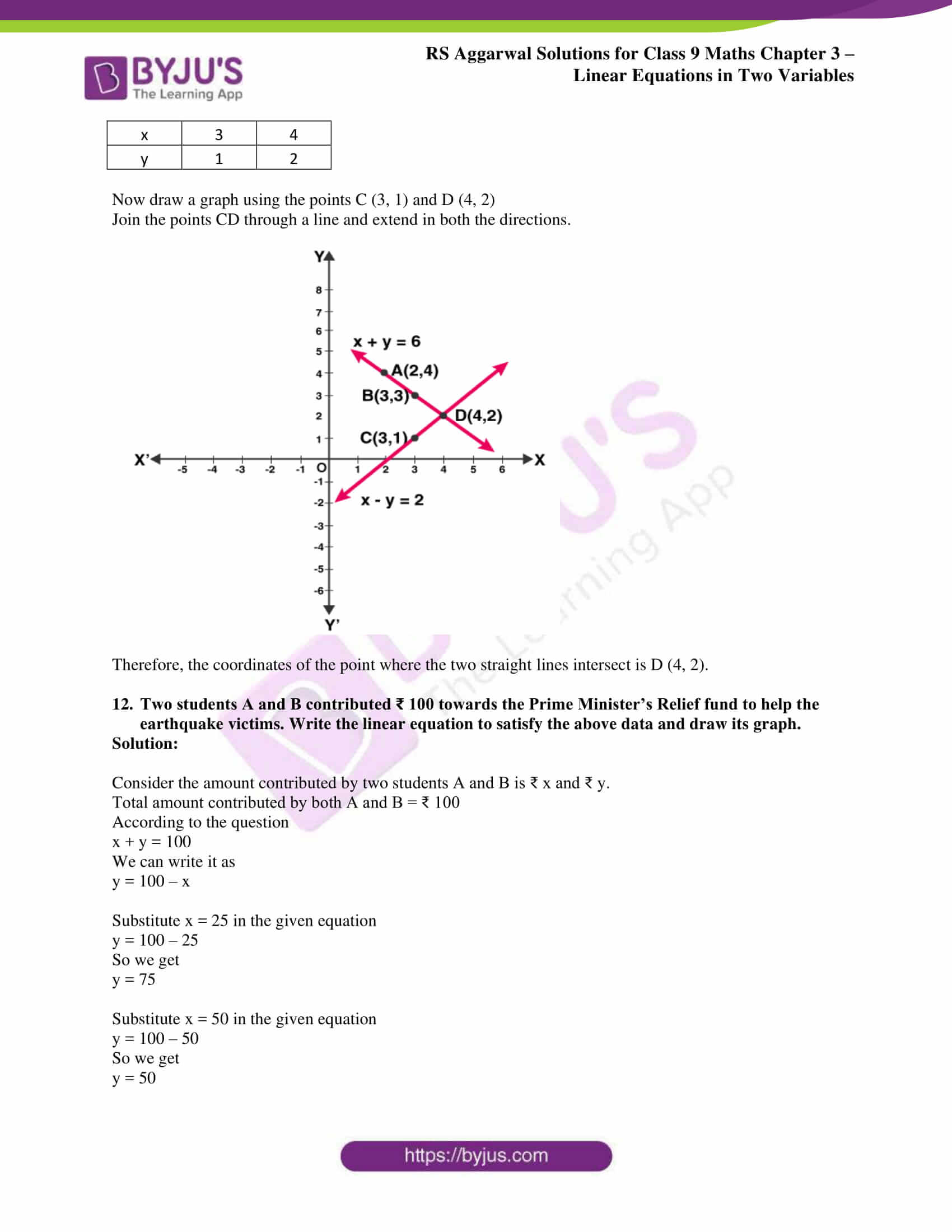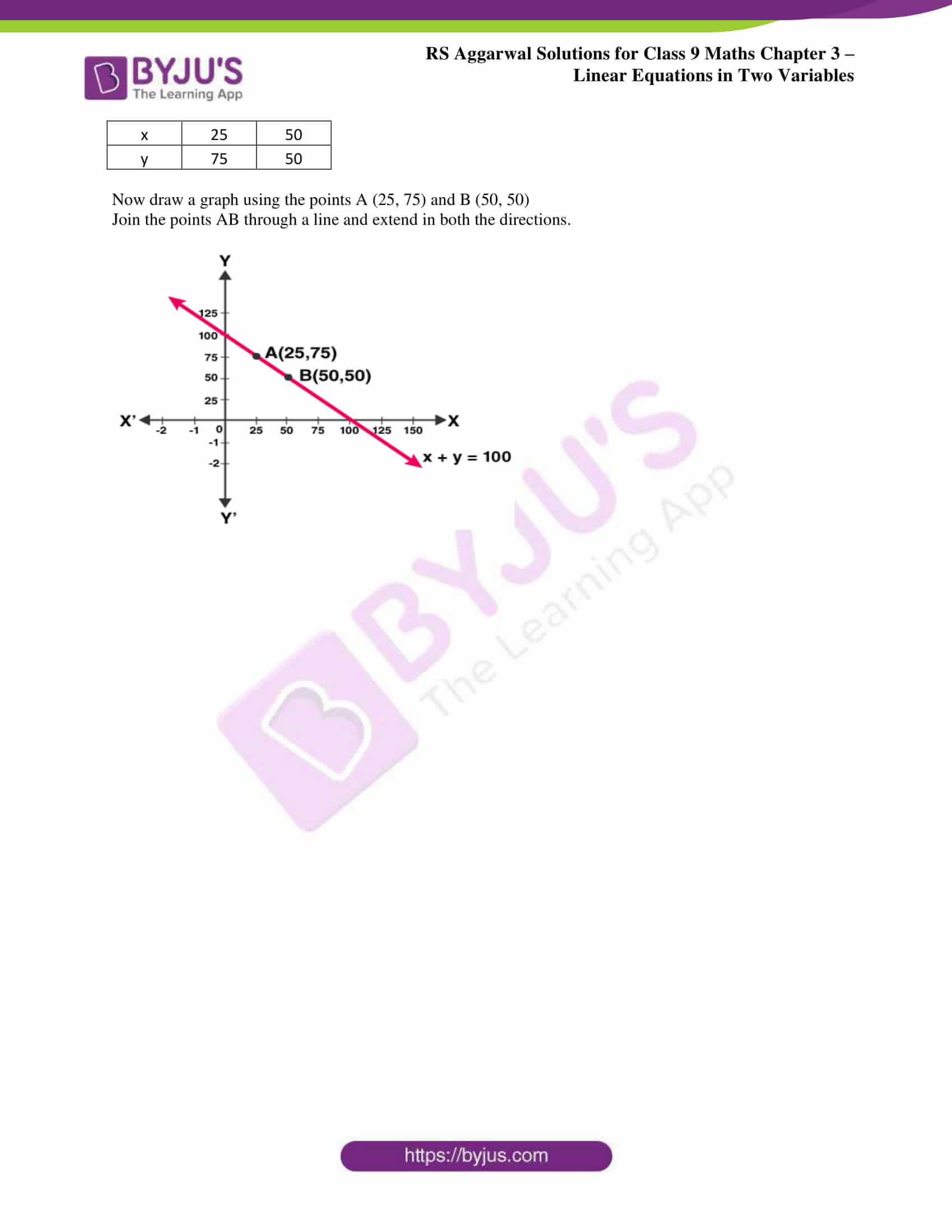### Access other exercise solutions of Class 9 Maths Chapter 4: Linear Equations in Two Variables

Exercise 4A Solutions 7 Questions

### RS Aggarwal Solutions Class 9 Maths Chapter 4 – Linear Equations in Two Variables Exercise 4B

RS Aggarwal Solutions Class 9 Maths Chapter 4 Linear Equations in Two Variables Exercise 4B covers the topic Graph of a Linear Equation in Two Variables with set of examples to provide better understanding of the concepts among the students.

### Key features of RS Aggarwal Solutions for Class 9 Maths Chapter 4: Linear Equations in Two Variables Exercise 4B

• The main vision of providing solutions in PDF is to improve the grasping abilities among the students.
• The step wise problems solved by experts mainly improves time management which is a critical aspect in exams.
• The solutions are a perfect source of reference and can be used as a comprehensive study material for exam preparation.
• Self analysis can be done by students to understand the mistakes they conduct while solving to improve the time taken for solving each problem.